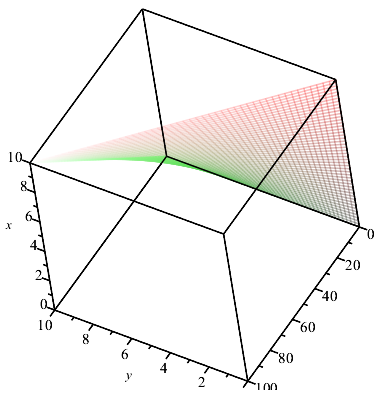## How to find endogenous variable over a set of two ...

Hello everyone,

I'm studying an equation with three variables similar to the following:Now, I would like to obtain the numeric values of x associated to (y, z) that are free to assume any integer value within the intervals [-1...5] and [0...5] respectively. Thus, I should get 42 values of x overall. The question is very similar to the one asked here: https://www.mapleprimes.com/questions/146636-How-To-Solve-An-Equation-In-Three-Variables but in my case I would like to obtain all the 42 values of x (included undefined solutions) associated to the sets (y,z), possibly arranged in a 42x3 matrix where the columns are the variables (x,y,z) and the rows the values (x,y,z) that satisfy the equation.

I started to tackle the problem using a for loop:

for y from -1 by 1 to 5 do

for z from 0 by 1 to 5 do

eq:= fsolve(x*y + z, x);

end do;

end do;

eq;

But it returns only one value: -1.000. Any help is appreciated.

## Difficulties in integrating and plotting...

I'm trying to plot this integral but without success. Could someone help me?  I tried it the following way.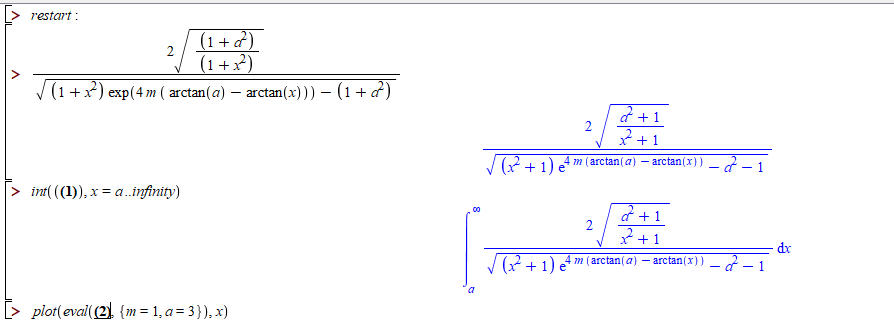Thanks in advance for any help!

integral_test.mw

## Solve is acting weird, giving too many solutions c...

Why on earth is Maple giving me all these solutions compared to last year??

Last year: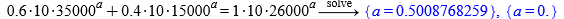Now: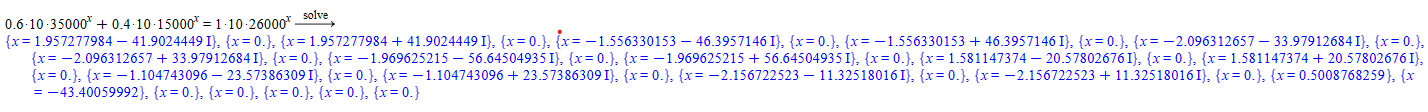## Where is the problem (Wrong or At Least Unexpected...

Hi all;

I hope all is well with you.

I am regular user of Maple for 7 years. I just reinstalled it to a new version, Maple 2019.

After installation, which was very smooth and without any unexpected problem, I opened a new document and entered a simple task; “sqrt(4);” the answer was

1.81847767202745*10^(-58) + (7.53238114626421*10^(-59))*I

I am pretty sure that I am missing something. The answer is obviously incorrect and there is a problem, but where.

I would be thankful if someone could help me with this issue.

Best

## Is it possible to show the values of time and a va...

Hi all,

I'm using dsolve (numeric) for a system of ODE with initial conditions.

Is it possible to show (at the same time with solving) the values of time and an unknown when solving a system of ODE before the end of calculation. Of course, the calculation time is high and I want to know before the calculation is finished if the solution is converging.

Also, I use the option range in my calculations from 0 to 200s (seconds) and more. I do'nt know how I can speed up the solution with less memory.

Please find in the attached file an example.

dsolve1_1r.mw

## A different kind of differentiation...

Hi folks!

Is there any way to make this kind of derivative?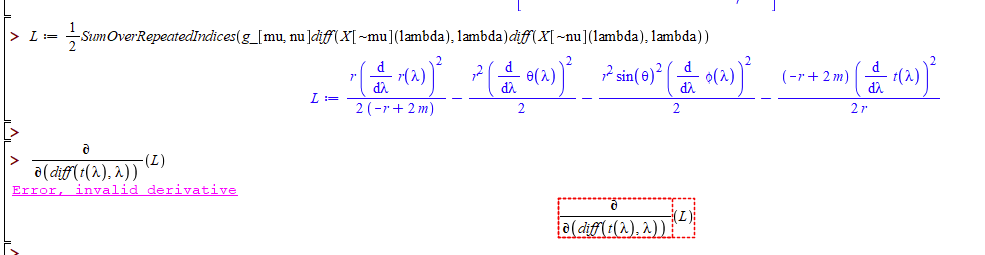If necessary, I can provide the original file here.

Thanks in advance for any help!

## shading, style and lightmodel oddities...

choosing lightmodel=none and shading=none, produces a dark grey grided surface
plot3d(x*y, x = 0 .. 10, y = 0 .. 10, lightmodel = none, shading = none)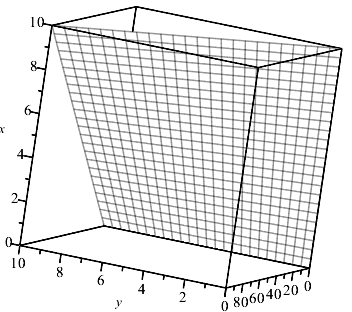adding the style=wireframe option gives a blank plot.  Grid probably white?  Changing style to patchnogrid the surface is indeed white. However chosing both shading and style options to none regardless of the lightmodel will produce a plot that appears empty.  Is this to be expected?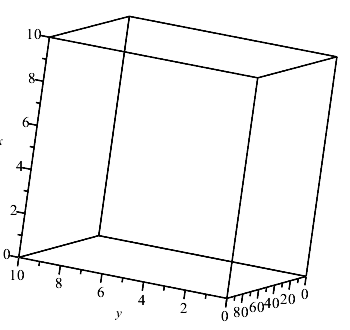However, just the style=wireframe option produces a colored grided wireframe as to expect
plot3d(x*y, x = 0 .. 10, y = 0 .. 10, lightmodel = none, style = wireframe)